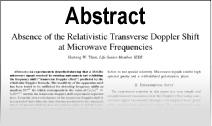Enter the content which will be displayed in sticky bar

AbstractPhysics ZMV; The Basis of New Physics

Year: 2018 Pages: 19

The Beginning of all Origins is the gravitational field. It is formed from gravitons in motion. The graviton is an elementary quantum of energy when it is in the state of motion and an indivisible particle of mass when it is in the state of rest. Physics ZMV is based on the idea of the existence of the gravitational field independent of the mass and the mass independent of the gravitational field. The potential energy of the gravitational field is directly proportional to the difference between the gravitational field energy that enters in the mass and emerges from the mass. The masses are not gravitationally attracted to each other. The potential energies of the gravitational field compress the masses to their common centre of mass. Compression of masses by the potential energies of the gravitational field is the first fundamental law of Physics ZMV. The mass is a conglomerate of gravitons in the state of rest; that is, the gravitons which have given the mass its kinetic energy and remained in the mass (increasing the amount of the mass). The mass is heated by this energy. Heating of the mass by the gravitational field is the second fundamental law of Physics ZMV. Growth of the mass by the gravitational field is the third fundamental law of Physics ZMV. The mass motion of inertia is the mass motion under the action of the inertia energy of the gravitational field. Mass motion by inertia energy of the gravitational field is the fourth fundamental law of Physics ZMV. The mass which received rectilinear and rotational motion (rotational axis is perpendicular to the vector of rectilinear speed) changes the volume of the mass parts in synchronous with the angular motion. This phenomenon is named flux and re-flux of the mass. Flux and re-flux of the mass is the fifth fundamental law of Physics ZMV. The category \"space\" and the category \"ether\" have the same physical meaning - are the receptacle of moving and resting gravitons. The ether consists of etherons, that is, the space consists of etherons. Etheron is the minimal, indivisible cell of space (ether). All the masses in the Universe (from micro particles to black holes) in its past were a gravitational field, that is, moving gravitons in space (ether). The energy of a homogeneous gravitational field is a \"dark energy\". The mass of a homogeneous gravitational field (because gravitons move) is a \"dark mass\". The energy - is the moving mass. The mass - is the resting energy. The speed at which the mass is moving under the action of a force in space without a gravitational field is directly proportional to the force and inversely proportional to the mass: F = zm V, where z - is a coefficient of proportionality. That is why this physics is named Physics ZMV.